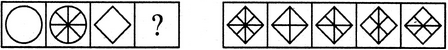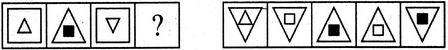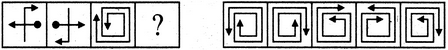# Non Verbal Reasoning - Analogy

Exercise : Analogy - Section 1
Directions to Solve

Each of the following questions consists of two sets of figures. Figures A, B, C and D constitute the Problem Set while figures 1, 2, 3, 4 and 5 constitute the Answer Set. There is a definite relationship between figures A and B. Establish a similar relationship between figures C and D by selecting a suitable figure from the Answer Set that would replace the question mark (?) in fig. (D).

6.

Select a suitable figure from the Answer Figures that would replace the question mark (?).(A)     (B)      (C)     (D)                  (1)      (2)      (3)      (4)      (5)
1
2
3
4
5
Explanation:
The combination of two symbols placed at the lower-right corner, rotates 90oCW and moves to the Upper-right corner. Also, the combination of two symbols placed at the upper-left corner, moves to the lower-right corner.

7.

Select a suitable figure from the Answer Figures that would replace the question mark (?).(A)     (B)      (C)     (D)                  (1)      (2)      (3)      (4)      (5)
1
2
3
4
5
Explanation:
The figure gets divided into eight equal parts.

8.

Select a suitable figure from the Answer Figures that would replace the question mark (?).(A)     (B)      (C)     (D)                  (1)      (2)      (3)      (4)      (5)
1
2
3
4
5
Explanation:
The inner element enlarges to become the outer element while the outer element reduces in size, turns black and becomes the inner element.

9.

Select a suitable figure from the Answer Figures that would replace the question mark (?).(A)     (B)      (C)     (D)                  (1)      (2)      (3)      (4)      (5)
1
2
3
4
5
Explanation:
The figure rotates through 90oACW and the arrowhead shifts closer to the black circle.

10.

Select a suitable figure from the Answer Figures that would replace the question mark (?).